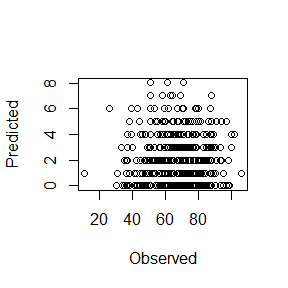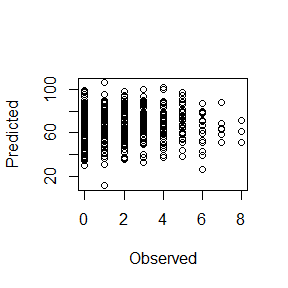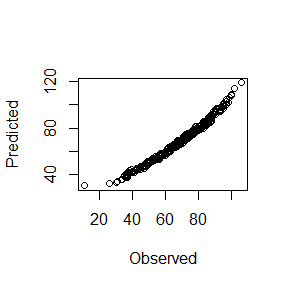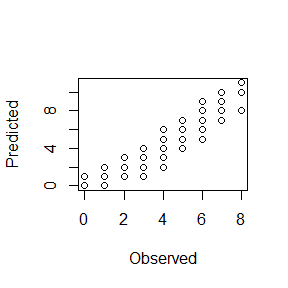# Gaussian and Poisson CRFs

The theory behind using nodewise regression to approximate Markov Random Fields and Conditional Random Fields models was developed with binary presence-absence data in mind. However, the same principle can hold for other data structures, as long as we recognise the key limitations. For Gaussian and Poisson variables, the primary issue with fitting MRF or CRF models in this fashion is that the outcome variables are not necessarily on the same scale. Consider a community where we have three species that show some associations in their abundances, but the ranges of these abundances are quite different (this could perhaps apply to a quickly-reproducing prey species and some wide-ranging predator species, for example). Here, we simulate a dataset with two fake covariates and three species to illustrate the problem

cov <- rnorm(500, 0.2)
cov2 <- rnorm(500, 4)
sp.2 <- ceiling(rnorm(500, 1) + (cov * 2))
sp.2[sp.2 < 0] <- 0
poiss.dat <- data.frame(sp.1 = ceiling(rnorm(500, 4) + (cov2 * 15.5) + (sp.2 * -0.15)),
sp.2 = sp.2, sp.3 = ceiling((sp.2 * 1.5) + rnorm(500, 0.1)))
poiss.dat[poiss.dat < 0] <- 0
poiss.dat$cov <- cov poiss.dat$cov2 <- cov2

By investigating the ranges of the species’ abundance vectors, we can see that they are quite different, with sp.1 generally showing higher abundance than the other two species

apply(poiss.dat[, c(1:3)], 2, range)
##      sp.1 sp.2 sp.3
## [1,]   11    0    0
## [2,]  106    8   13

This can cause a problem for fitting MRF / CRF models, as we are essentially regressing each species’ abundance against the abundances of all other species (and covariates) and then symmetrising the respective parameter estimates (taking the mean of the two estimates, by default). This will undoubtedly lead to severe overestimates for the species with lower abundance ranges and underestimates for the common species. We can illustrate this here for sp.1 and sp.2 by running a simple glm for each species by including the other species as a predictor

sp.1.vs.sp.2 <- coef(glm(sp.1 ~ sp.2, family = 'poisson', data = poiss.dat))
sp.2.vs.sp.1 <- coef(glm(sp.2 ~ sp.1, family = 'poisson', data = poiss.dat))
mean.coef <- mean(sp.1.vs.sp.2, sp.2.vs.sp.1)

# Exponentiate, due to the log link
mean.coef <- exp(mean.coef)

# Make plots of predicted vs observed
# First, predict sp.1 (the common species) abundances
plot(poiss.dat$sp.1, poiss.dat$sp.2 * mean.coef,
xlab = 'Observed',
ylab = 'Predicted')# Now predict sp.2 (the more rare species) abundances
plot(poiss.dat$sp.2, poiss.dat$sp.1 * mean.coef,
xlab = 'Observed',
ylab = 'Predicted')To overcome the issue of possible differences in ranges for Poisson variables, MRFcov uses a nonparanormal transformation method whereby each variable is log2(x + 0.1) transformed and mapped to a normal distribution via a copula approach prior to fitting the model(see here for more information). The model is then fitted by considering the variables as Gaussian (e.g., there is no link function for these models). In doing so, the variables are all normalised without adding too much noise (though this transformation should always be recognised as a limitation). Any Poisson models that are fit will return an additional item called poiss_sc_factors, which will be necessary to back-transform outputs back to the variables’ original distributions. This is done by estimating each variable’s negative binomial or poisson parameters, depending on which distribution was more appropriate

library(MRFcov)
poiss.crf <- MRFcov(data = poiss.dat, n_nodes = 3, family = 'poisson')
## Poisson variables will be transformed using a nonparanormal...
## Fitting MRF models in sequence using 1 core ...
poiss.crf$poiss_sc_factors ## sp.1 sp.2 sp.3 ## size 27.27841 2.315283 2.412597 ## mu 66.68800 2.081999 3.784001 Our coefficient for the effect of cov2 on sp.1 above was 15.5. To see how the model did in isolating this effect, we can get an approximation by converting the effect using the sd of the raw variable (this is because the model was fitted to normalised variables that were at unit variance) sd(poiss.dat[,1]) * poiss.crf$direct_coefs$cov2 ##  15.27594 Not bad, but again this is an approximation. However for generating predictions, MRFcov will first generate the normalised predictions and then map these to each raw variable’s appropriate distribution (either negative binomial or poisson, depending on which was more appropriate). This is a better way to ensure that outcomes are converted to the correct range. For example, we can use our fitted model to predict the raw data and generate similar plots to those that we created above poiss.preds <- predict_MRF(data = poiss.dat, MRF_mod = poiss.crf, n_cores = 1) plot(poiss.dat$sp.1, poiss.preds[,1],
xlab = 'Observed',
ylab = 'Predicted')plot(poiss.dat$sp.2, poiss.preds[,2], xlab = 'Observed', ylab = 'Predicted')These plots should look much better. We can also inspect the outputs of the cv functions for Poisson data. MRFcov can use cross-validation (fitting models to specific subsets of the data and using resulting equations to predict observations for the remaining subset) to describe model fit and compare models with and without covariates. For family = binomial models, as seen at the end of the MRFs and CRFs for Bird.parasites data vignette, the functions return information on classification accuracy. But for Poisson data, it instead returns information on model deviance and mean squared error. We can showcase this functionality here using the fake data we have generated. We would expect that a CRF would fit the data better in this case, as this model includes the two covariates that we know are influential poiss.cv <- cv_MRF_diag(data = poiss.dat, n_nodes = 3, n_folds = 5, n_cores = 1, family = 'poisson', compare_null = TRUE, plot = FALSE) ## Generating node-optimised Conditional Random Fields model ## Poisson variables will be transformed using a nonparanormal... ## Fitting MRF models in sequence using 1 core ... ## ## Generating Markov Random Fields model (no covariates) ## Poisson variables will be transformed using a nonparanormal... ## Fitting MRF models in sequence using 1 core ... # CRF (with covariates) model deviance range(poiss.cv$Deviance[poiss.cv$model == 'CRF']) ##  5.085791 43.327080 # MRF (no covariates) model deviance range(poiss.cv$Deviance[poiss.cv\$model != 'CRF'])
##  174.1158 281.6758

Lower deviance for the CRF model confirms the idea that inclusion of covariates is supported.

Please note that the type of normalisation used above only applies to Poisson models!! Gaussian variables are not standardised before fitting CRFs / MRFs, so users should inspect the ranges of their variables and consider using the scale function to standardise beforehand if need be. The reason Gaussian models do not do this by default is that there are many options for normalisation / standardisation for Gaussian data and so users may wish to have more control over how this is done before fitting models